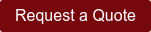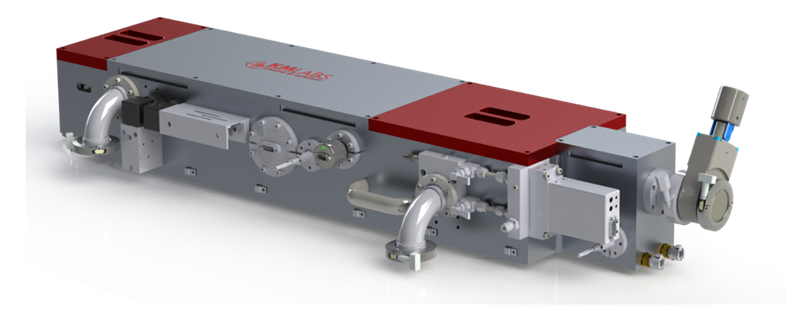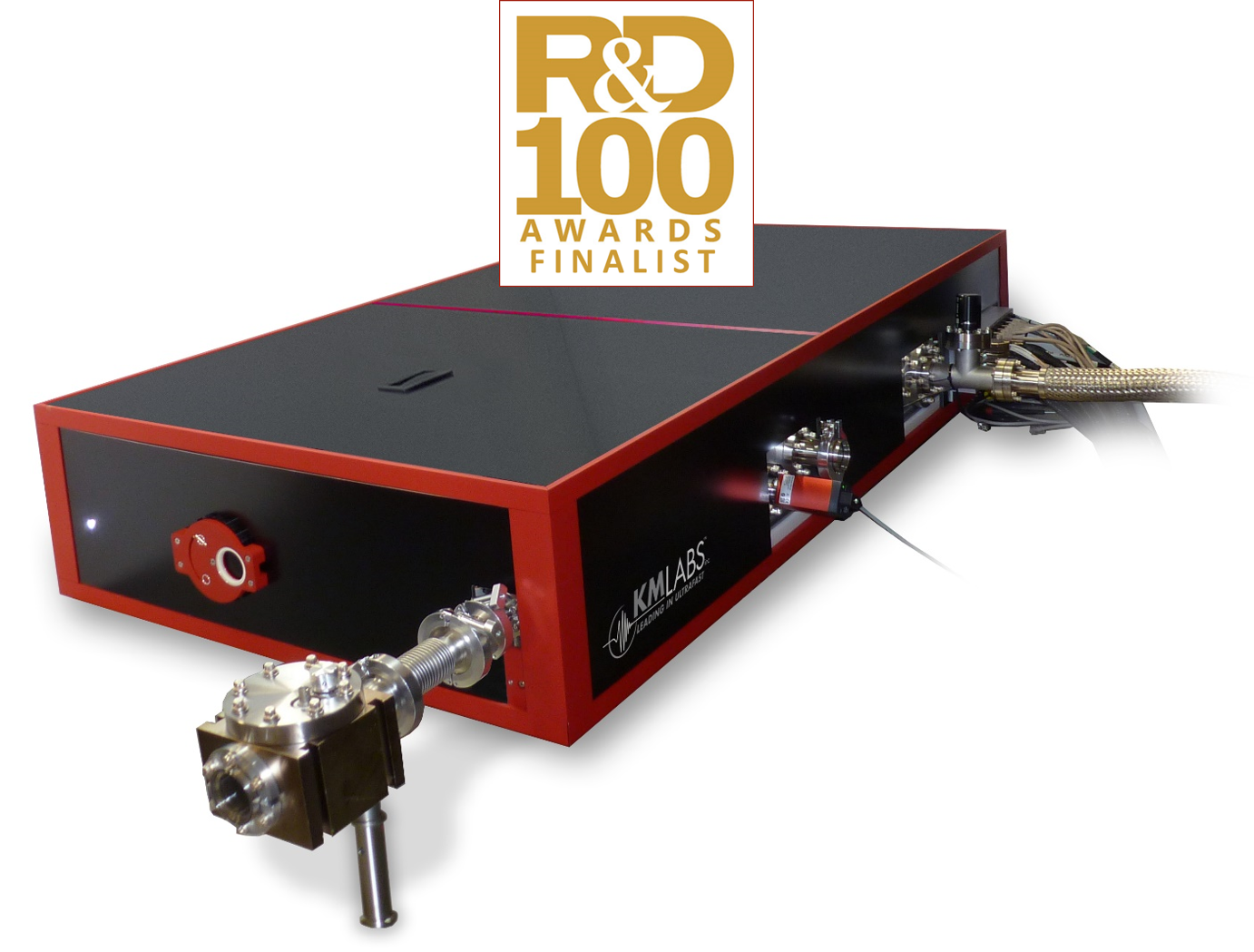### Easy to use calculator!

Need a quick unit conversion from wavelength (nm) to photon energy (eV)?  Need to convert flux (ph/s) to average power (mW)?  Simply enter the known value in the boxes below, and the calculator will provide the equivalent value in the appropriate unit.

## Calculation Theory

### Photon Energy and Wavelength

The energy of light (or photon energy), E, is inversely proportional to the wavelength by the equation:

E = hc/l     (equation 1)

where h is Planck's constant (6.6261 x 10-34 J*s) and c is the speed of light (2.9979 x 108 m/s).

Photon energy can be expressed using any unit of energy such as the electronvolt (eV) or the Joule (J). For short wavelength sources in the EUV to X-ray range, researchers often discuss photon energies in units of eV (or keV for hard X-rays) out of convenience.  The International System of Quantities (SI) have defined 1 eV to the exact value of 1.602176634×10−19 J.  Thus, equation 1 above can be rewritten for photon energy in eV and wavelength in nm as:

E (eV) = 1239.8 / l (nm)     (equation 2)

### Flux and Power

Power (P) is the rate of energy transfer with the SI unit of 1 Watt = 1 J/s.  The power of short wavelength light sources in the EUV to X-ray range are often expressed as photon flux (F) in the units of photons per second (ph/s).  Average power is related to flux and photon energy by:

P = F × E     (equation 3)

P = F × hc/l     (equation 4)

P (mW) = 1.989 × 10-10 × F/l (nm)     (equation 5)

For any given flux and wavelength (in nm), equation 5 can be used to calculate the corresponding average power (in mW).

## EUV and VUV Lasers

### Bring short wavelengths to your lab!

Now that VUV to EUV to SXR photons are becoming more readily available to everyday Physicists and Chemists, what ideas do you have in mind for your research??   Check out our Applications Page, to see what others are doing...

Compact 13.5 nm LaserAchieve the highest 13.5 nm flux on a tabletop scale.  Click here to learn more about the new XUUS 5 high harmonic generation source.

Hyperion VUV: 100 - 1030 nmA soft ionization source with tunable wavelengths at MHz repetition rates.  Ideal laser source for time-resolved spectroscopies and optical imaging applications.  Click here to learn more.